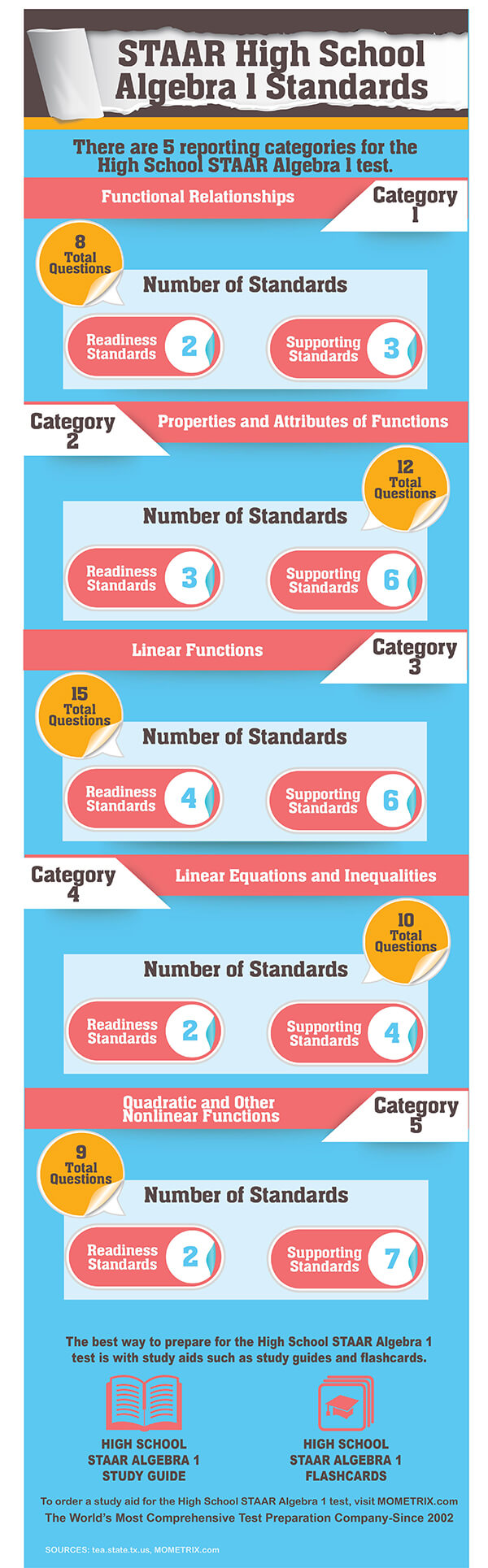# STAAR Algebra 1 Practice Test Questions

1. 25
2. 60
3. 12
4. 5
##### 2. Mr. Robinson has 20 students in his martial arts class. The ratio of boys to girls is 4:1. How many boys and girls are in Mr. Robinson’s class?
1. 15 boys, 5 girls
2. 5 boys, 15 girls
3. 16 boys, 4 girls
4. 4 boys, 16 girls
##### 3. Which of the following equations is an example of the distributive property?
1. (5)(3) = (3)(5)
2. 5+3 = 3+5
3. (5)(1+2) = (5)(1) + (5)(2)
4. 15=15
##### 4. Which of the following equations is an example of the commutative property?
1. (3)(6+10) = 18 + 30
2. 18 + 30 = 30 + 18
3. (3)(6) + (3)(10) = 3(16)
4. 48 = 48
##### What are the y-intercept and slope of the line?
1. The y-intercept is 40, and the slope is 10
2. The y-intercept is 10, and the slope is 40
3. The y-intercept is 40, and the slope is 2
4. The y-intercept is 4, and the slope is 0.5
##### 6. Line G has a slope of 20 and intercepts the y axis at point (0, 100). What is the equation of line G?
1. y = 100
2. y = 20
3. y = 20x – 100
4. y = 20x + 100
##### 7. Vivian wants to plant a vegetable garden that contains only tomatoes and cucumbers. However, she has a limited amount of space for the garden, and she can only afford to buy a specific number of each vegetable. Vivian has enough space to plant a total of 40 vegetables, and she has a total of \$80 to purchase the vegetables. Tomatoes cost \$1 per plant and cucumbers cost \$3 per plant. Let T represent the number of tomatoes and let C represent the number of cucumbers Vivian will plant in her garden.Which system of linear equations can be used to solve for the number of tomatoes and cucumbers Vivian will plant in her garden?
1. T + C = 40 and T + 3C = 80
2. T + 3C = 40 and T + C = 80
3. T + C = 80 and T + 3C = 40
4. 3T + C = 80 and T + C = 40
##### 8. {(5,8), (3,4), (-1,-4), (-3, -8), (-5,-12)}What is the range of the coordinate pairs?
1. {5, 8, -5, -12}
2. {-1, -4}
3. {-5, -3, -1, 3, 5}
4. {-12, -8, -4, 4, 8}
1. 4x < 30
2. 4x < 92
3. 4x > 30
4. 4x > 92
##### 10. Aisha runs a small business selling candy bars to her classmates in school. She buys each candy bar for \$0.75, and she sells each candy bar for \$1.50. Let y represent Aisha’s profit. Let x represent the number of candy bars she sells per day.Which equation best represents Aisha’s daily profit from selling candy bars?
1. y = 0.75x – 1.50x
2. y = 0.75x + 0.75x
3. y = 1.50x + 0.75x
4. y = 1.50x – 0.75x

The equation describes a functional relationship between x and f(x). To solve the equation, substitute 10 as the value of x, such that f(10)=5(10)+10=50+10=60.

Let = the number of girls in Mr. Robinson’s class. The ratio of boys to girls is 4:1. So for every 1 girl in the class, there are 4 boys in the class. Therefore, 4y equals the number of boys in Mr. Robinson’s class. The total number of students in the class is 20. Therefore, the number of boys plus the number of girls equals 20 or
y+4y=20
5y=20
y=4

Hence y = 4 and 4y = 16. Therefore, 4 = the number of girls and 16 = the number of boys. Also, 4 + 16 = 20, the total number of students in the class.

The distributive property says that terms inside a set of parentheses can be multiplied by a factor outside the parentheses. In other words, a(b+c) = ab + ac. Answer C fits this definition.

A mathematical operation is commutative if altering the order does not alter the result of the operation. In other words, a+b = b+a, or ab = ba. Answer B fits this definition.

First write Equation A in slope-intercept form: y =mx+b where b is the y-intercept and m is the slope:
10 y – 5x = 40
10 y = 5x + 40
y = 0.5x + 4
Based on the slope-intercept form of Equation A, the y-intercept b = 4, and the slope m = 0.5.

Write the equation in slope-intercept form: y = mx+b where m is the slope of the line and b is the y-intercept. In this case, the slope m = 20 and the y-intercept b = 100. Hence y = 20x+1000.